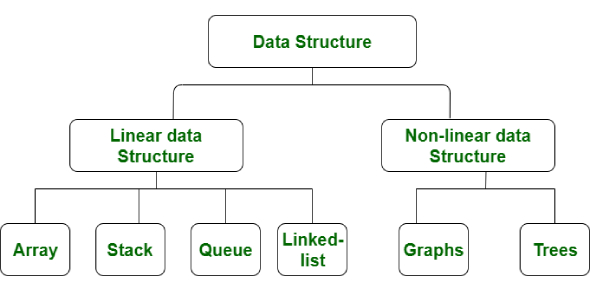# Data Structures Algorithms Online Quiz

41 Questions | Total Attempts: 773Settings.

• 1.
• A.

For relatively permanent collections of data

• B.

For the size of the structure and the data in the structure are constantly changing

• C.

For both of above situation

• D.

For none of above situation

• 2.
The complexity of merge sort algorithm is
• A.

.    O(n)

• B.

O(log n)

• C.

O(n2)

• D.

O(n log n)

• 3.
Which of the following data structure is not linear data structure?
• A.

Arrays

• B.

• C.

Both of above

• D.

None of above

• 4.
• A.

Components are all linked together in some sequential manner.

• B.

There is no beginning and no end.

• C.

Components are arranged hierarchically

• D.

Forward and backward traversal within the list is permitted.

• 5.
A linear collection of data elements where the linear node is given by means of pointer is called?
• A.

• B.

Node list

• C.

Primitive list

• D.

None

• 6.
Which of the following operations is performed more efficiently by doubly linked list than by singly linked list?
• A.

Deleting a node whose location in given

• B.

Searching of an unsorted list for a given item

• C.

Inverting a node after the node with given location

• D.

Traversing a list to process each node

• 7.
In linked list each node contain minimum of two fields. One field is data field to store the data second field is?
• A.

Pointer to character

• B.

Pointer to integer

• C.

Pointer to node

• D.

Node

• 8.
Consider the following definition in c programming language struct node { int data; struct node * next; } typedef struct node NODE; NODE *ptr; Which of the following c code is used to create new node?
• A.

Ptr=(NODE*)malloc(sizeof(NODE));

• B.

Ptr=(NODE*)malloc(NODE);

• C.

Ptr=(NODE*)malloc(sizeof(NODE*));

• D.

Ptr=(NODE)malloc(sizeof(NODE));

• 9.
A variant of linked list in which last node of the list points to the first node of the list is?
• A.

• B.

• C.

• D.

• 10.
What kind of linked list is best to answer question like “What is the item at position n?”
• A.

• B.

• C.

• D.

• 11.
......... form of access is used to add and remove nodes from a queue.
• A.

LIFO, Last In First Out

• B.

FIFO, First In First Out

• C.

Both a and b

• D.

None of these

• 12.
In liked representation of stack ....... holds the elements of the stack.
• A.

INFO fields

• B.

TOP fields

• C.

• D.

NULL fields

• 13.
........ form of access is used to add remove nodes from a stack.
• A.

. LIFO

• B.

FIFO

• C.

Both A and B

• D.

None of these

• 14.
New nodes are added to the ......... of the queue
• A.

. Front

• B.

. Back

• C.

Middle

• D.

Both A and B

• 15.
In the linked representation of the stack ......... behaves as the top pointer variable of stack.
• A.

Stop pointer

• B.

Begin pointer

• C.

. Start pointer

• D.

Avail pointer

• 16.
In linked representation of stack the null pointer of the last node in the list signals ..........
• A.

Beginning of the stack

• B.

Bottom of the stack

• C.

Middle of the stack

• D.

In between some value

• 17.
What happens when you push a new node onto a stack?
• A.

The new node is placed at the front of the linked list

• B.

The new node is placed at the back of the linked list

• C.

The new node is placed at the middle of the linked list

• D.

No Changes happens

• 18.
A queue is a .........
• A.

FIFO

• B.

. LIFO

• C.

FILO

• D.

FILO

• 19.
Which of the following name does not relate to stacks?
• A.

. FIFO lists

• B.

LIFO lists

• C.

Piles

• D.

Push down lists

• 20.
The retrieval of items in a stack is ........... operation.
• A.

. push

• B.

. pop

• C.

Retrieval

• D.

Access

• 21.
The term push and pop is related to
• A.

Array

• B.

Lists

• C.

. Stacks

• D.

Trees

• 22.
Which is the pointer associated with the stack?
• A.

FIRST

• B.

FRONT

• C.

TOP

• D.

REAR

• 23.
The elements are removal from a stack in .......... order.
• A.

Reverse

• B.

Hierarchical

• C.

Alternative

• D.

Sequential

• 24.
The insertion operation in the stack is called .........
• A.

Insert

• B.

Push

• C.

. pop

• D.

Top

• 25.
...... is the term used to insert an element into stack.
• A.

Push

• B.

. Pull

• C.

. Pop

• D.

Pump

Related TopicsBack to top